Home    |    Teacher    |    Parents    |    Glossary    |    About UsIn this lesson, we study some common space figures that are not polyhedra. These figures have some things in common with polyhedra, but they all have some curved surfaces, while the surfaces of a polyhedron are always flat.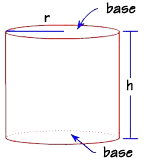First, the cylinder. The cylinder is somewhat like a prism. It has parallel congruent bases, but its bases are circles rather than polygons. You find the volume of a cylinder in the same way that you find the volume of a prism: it is the product of the base area times the height of the cylinder: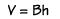Since the base of a cylinder is always a circle, we can substitute the formula for the area of a circle into the formula for the volume, like this: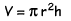Let's find the volume of this can of potato chips.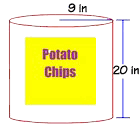We'll use 3.14 for pi. Then we perform the calculations like this: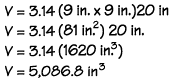That's a lot of potato chips!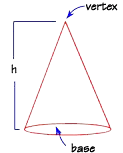A cone has a circular base and a vertex that is not on the base. Cones are similar in some ways to pyramids. They both have just one base and they converge to a point, the vertex.

The formula for the volume of a cone is: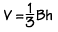Since the base area is a circle, again we can substitute the area formula for a circle into the volume formula, in place of the base area. The final formula for the volume of a cone is: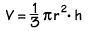Let's find the volume of this cone.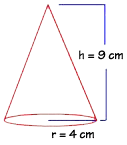We can substitute the values into the volume formula. When we perform the calculations, we find that the volume is 150.72 cubic centimeters.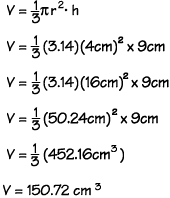Finally, we'll examine the sphere, a space shape defined by all the points that are the same distance from the center point. Like a circle, a sphere has a radius and a diameter. The shape of the earth is like a large sphere -- it has radius of about 4000 miles. A tennis ball is a sphere with a radius of about 2.5 inches.

Since a sphere is closely related to a circle, you won't be surprised to find that the number pi appears in the formula for its volume: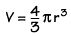Let's find the volume of this large sphere, with a radius of 13 feet. Notice that the radius is the only dimension we need in order to calculate the volume of a sphere.

If we substitute 13 feet for the radius, then we get 9,198.11 cubic feet.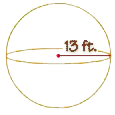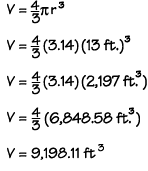Homework Help | Geometry | Three-dimensional figuresEmail this page to a friendSearch·  Space figures·  Prisms·  Pyramids·  Cylinders, cones     and spheresFirst Glance In Depth Examples WorkoutCylinders, cones and spheres